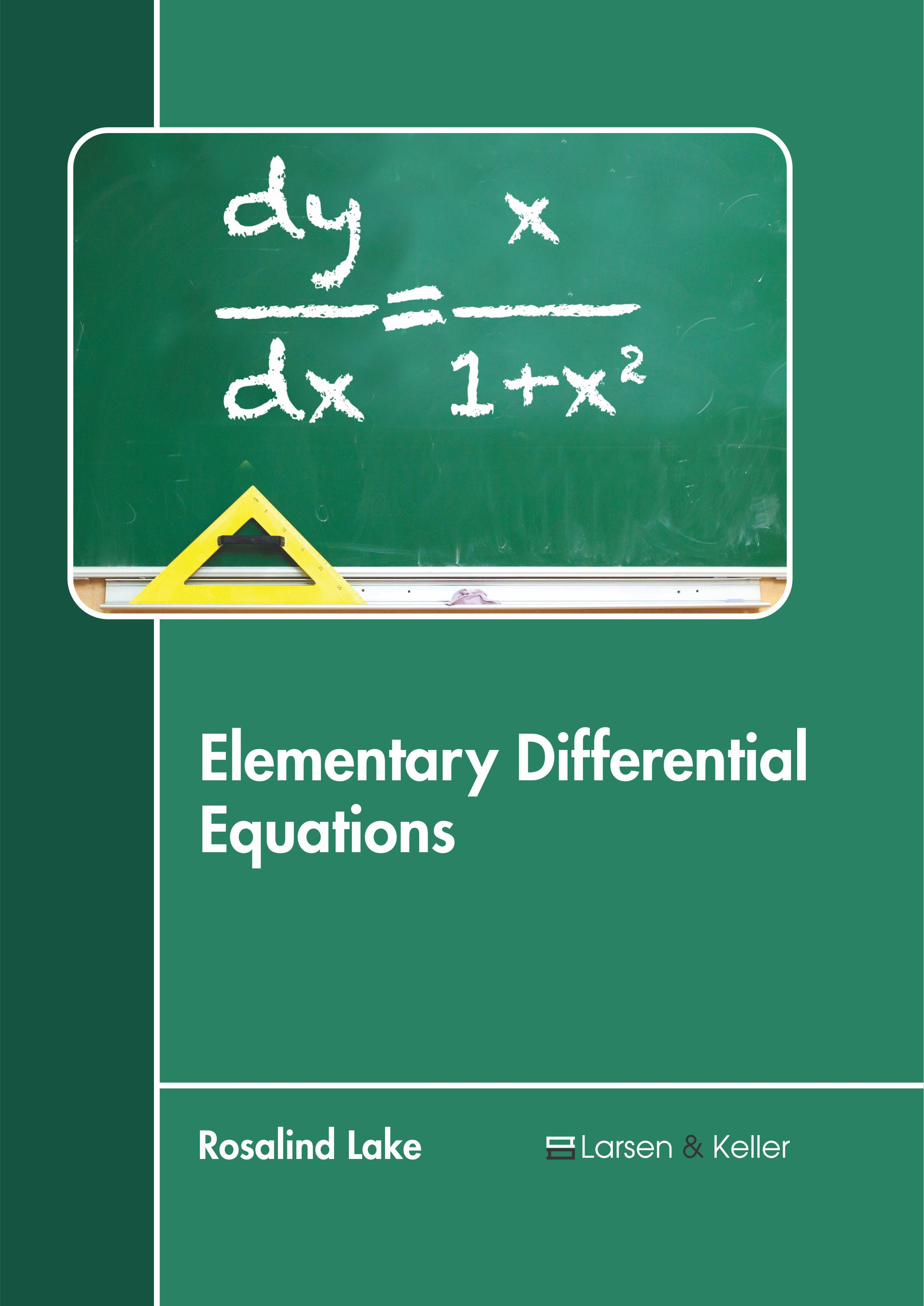# books

BROWSE BY

#### Author

Results 1 - 1 of 1Elementary Differential Equations Author : Rosalind Lake Subject : Mathematics and Statistics ISBN :9781641721219 A differential equation is a mathematical equation containing functions and their derivatives. A function represents a physical quantity, its derivative expresses the rate of change and the equation describes Read More
Results 1 - 1 of 1